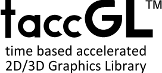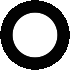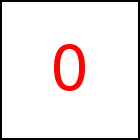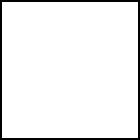# Simple WebGL™ Html5 Popup Animations with no more than 4 Lines of javaScript

Pop-Ups are very common in today's HTML5/CSS web pages, as explicit pop-up boxes, dialog or alert boxes, as pop-up menues and much more.

The first very simple example shows a pop-up flying in from the top left of the screen. WebGL™ would not have been necessary for this first example, just to show the basics. The example consists of taccgl.actor("popup",null,"visible"), which selects the HTML element with id=popup for animation and specifies "visible" to make this element visible after playing the animation. The from(0,-400,0) then defines a transition that moves the pop-up into the page from coordinates (0,-400) i.e. the top left of the screen. from works in 3D and needs a third coordinate z=0. The duration(2) defines that the transition will run for 2 seconds. After being stated the animation runs in parallel to further execution of javaScript.

 taccgl.actor("popup",null,"visible"). from(0,-400,0) . duration(2) . start() RUN

For a detailed description of this javaScript code please refert to the taccgl™ Tutorial.

The next simple example moves in a box. It differs from the first example by using taccgl.dddBox as second parameter for actor (Boxes). Using depth(200) the depth of the box is specified. The rotateMiddle specifies an additional rotation. Rotations will be discussed below. See Simple Rotation.

 taccgl.actor("popup",taccgl.dddBox,"visible"). depth(200). from(-800,800,0). rotateMiddle(0.7,0.7,0). duration(2).start() RUN

All the examples on this page open and close a sample text box that is formatted using HTML and CSS as follows:



## Continuation

The popup-animations in this article are intended to play when an HTML element changes from visibility:hidden to visibility:visible. During the animation the element is still hidden and the animation is displayed with WebGL™; after the animation WebGL™ becomes inactive and the element visible. So in the box example above the top and right sides of the box vanish when the animation is finished. The following example helps to further smooth this transion. See .

Using cont multiple transitions can be concatinated. The following example first moves in the box as in the example above and then flattens the box. Using cont this second flattening step is defined. depth(600,1) defines a transition gradually reducing the size of the box from 600 to 1. See Continuations.

 taccgl.actor("popup",taccgl.dddBox,"visible"). from(-800,800,0).depth(600). rotateMiddle(0,.7,.7).duration(2).start().cont().depth(600,1).dur(2).start() RUN

## Resizing and Flexibles

Let's first start with two examples using resize. resize defines an animation that gradually grows or shrinks the animated element. In the example below resize(1,a.h,a.w,a.h) starts with the element of width 1 and its normal height (a.h) and grows it to its normal width (a.w) and height (a.h). This example uses expressions as discussed in Expressions. The second example in addition to the same resize operation moves the element from left to right, so that the element seems opening in the middle.

 (a=taccgl.actor("popup",null,"visible")) . resize(0.1,a.h,a.w,a.h) .duration(1).start() RUN (a=taccgl.actor("popup",null,"visible")) . from (a.x+a.w/2,a.y,0). resize(1,a.h,a.w,a.h) .duration(1).start() RUN

The following examples, in addition to resize, change the shape of the element: First flexiBorder is used to make the element flexible. Then Wave is used to give the element a Wave form. Finally Rect1 is used to gradually change the Wave form into the normal rectangular form.

 (a=taccgl.actor("popup",taccgl.flexiBorder,"visible")) .resize(0.1,a.h,a.w,a.h) .Wave(40,40,-3.14159,3.14159) .Rect1() .duration(1) .start() RUN (a=taccgl.actor("popup",taccgl.flexiBorder,"visible")) .from (a.x+a.w/2,a.y,0) .resize(1,a.h,a.w,a.h) .Wave(40,40,-3.14159,3.14159) .Rect1() .duration(1) .start() RUN (a=taccgl.actor("popup",taccgl.flexiBorder,"visible")) .resize(0.1,a.h,a.w,a.h) .ZWave(0.2,0.2,-3.14159,3.14159) .Rect1() .duration(1) .start() RUN (a=taccgl.actor("popup",taccgl.flexiBorder,"visible")) .from (a.x+a.w/2,a.y,0) .resize(1,a.h,a.w,a.h) .ZWave(0.2,0.2,-3.14159,3.14159) .Rect1() .blend(0,0,1,0) .duration(1) .start() RUN

The previous 2 examples in addition use ZWave to create a 3D-Wave form and blend to fade in the element.

The next example works in two steps. First it gives the element a circle form and grows it from one pixel size up to a full circle. Then in a second step after the cont it gradually transforms the element from circle shape into the usual rectangular shape. See Flexibles.

 (a=taccgl.actor("popup",taccgl.flexiBorder,"visible")) . from (a.x+(a.w-a.h),a.y+a.h/2,0).to (a.x+(a.w-a.h)/2,a.y,0) .resize(1,1,a.h,a.h) .Circle() .duration(1) .start() .cont() .Rect1() .flyHome().resize(a.h,a.h,a.w,a.h) .start() RUN

## Acceleration

The following 3 examples use vEnd(0,0,0) to set the final velocity to 0, which means the motion gradually slows down. The second and third example show a motion from top and from left, by setting the starting coordinates differently using the x and y coordinates of the HTML element a.x and a.y. See Accelerated Motion.

 taccgl.actor("popup",null,"visible").from(0,-400,0) .vEnd(0,0,0) .duration(2).start() RUN (a=taccgl.actor("popup",null,"visible")) .from(a.x,-400,0) .vEnd(0,0,0) .duration(2).start() RUN (a=taccgl.actor("popup",null,"visible")) .from(-400,a.y,0) .vBegin(1500,500,0) .duration(2).start() RUN (a=taccgl.actor("popup",null,"visible")) .from(-400,a.y,2000) .vBegin(1500,500,0) .duration(2).start() RUN (a=taccgl.actor("popup",null,"visible")) .from(-00,a.y,-2000). vEnd(0,0,0) .duration(2) .start() RUN

## Rotation

The next two examples add some rotation using rotateMiddle. The parameters specify an axis for the rotation. So rotateMiddle(0,1,0) rotates around the y-axis and rotateMiddle(0,.7,.7) around the diagonal of the y and z axis. See Simple Rotation.

 taccgl.actor("popup",null,"visible").from(0,1000,0).rotateMiddle(0,1,0) .vEnd(0,0,0) .duration(3) .start() RUN (a=taccgl.actor("popup",null,"visible")) .from(-500,a.y,0) .rotateMiddle(0,.7,.7) .vEnd(0,0,0) .duration(3) .start() RUN (a=taccgl.actor("popup",taccgl.dddBox,"visible")) .from(a.x,1200,0) .depth(250,250).rotateMiddle(1,0,0) .rotatePart(0,-Math.PI*2) .duration(2) .start(). cont() .depth(250,1) .start() RUN (a=taccgl.actor("popup",taccgl.dddBox,"visible")) .from(-500,a.y-500,0) .depth(600) .rotateMiddle(0,.7,.7).vEnd(0,0,0) .duration(3) .start() .cont() .depth(600,1) .start() RUN

taccgl™ can automatically show shadows casted from animated elements / objects on other animated elements / objects. In above examples, there are no shadows, because there is just one animated element, while the main body of the page is just HTML and not an element animated with taccgl™. In the example below this is changed using taccgl.a(document.body), which makes the complete document body an animated element. color("black") makes it completely opaque and shadowOnly makes sure that only shadows appearing on the body are drawn with taccgl™, not the content of the body itself.

The next thing needed is posZ, e.g. posZ(-400). This places the pop-up box 400 pixels in front of the document body. With the default z coordinate of z=0, the pop-up box would be immediately on top of the body and consequently case no shadow on it.

Now you will notice on the first example below, that the pop-up shown is enlarged. This is, because it is 400px nearer to the camera and so appears larger. This perspective effect can be undone by resizing the element using resizeZ (see second example).

 taccgl.a(document.body).color("black") .shadowOnly() .dur(6) .start(); taccgl.actor("popup",null,"visible").posZ(400) .from(0,2000,400).rotateMiddle(0,1,0). vEnd(0,0,0).dur(4) .showAfter() .start() RUN taccgl.a(document.body) .color("black") .shadowOnly() .dur(6) .start(); taccgl.actor("popup",null,"visible").posZ(400) .resizeZ() .from(0,2000,400).rotateMiddle(0,1,0) .vEnd(0,0,0) .dur(4) .showAfter() .start() RUN

Finally you will notice that the shadow eventually disappears. This is the case because then the taccgl™ animation terminates and the pop-up box is displayed using HTML.

## Page Flip Animation

Another nice effect made with HMTL5/WebGL™ is a page flip lke animation which displays a curved surface.

 taccgl.a(document.body).color("black").shadowOnly().start(); taccgl.actor("popup",taccgl.flexiBorder,"visible") .flyIn(-600,4000,0). Flip(0,0). Rect1(). vEnd(0,0,0) . start() RUN

 taccgl.a(document.body).color("black").shadowOnly().dur(3).start(); taccgl.actor("popup",taccgl.flexiBorder,"visible") .flyIn(-600,1000,-3000). Flip(0,0). Rect1(). vEnd(0,0,0) . dur(3). start() RUN

## Multi Animations

Using sliceV an element can be cut into vertical slices. All methods called afterwards act separately on each of the slices in parallel.

sliceH cuts the element into horizontal slices and By using both sliceV and sliceH the element is cut into rectangular pieces. sequence is used to perform all the transitions in a sequence.

 taccgl.a(document.body).color("black") .shadowOnly() .dur(6) .start(); taccgl.ma("popup").paint() .posZ(400) .resizeZ() .visFinal('visible') .sliceV(10) .from(0,2000,400).rotateMiddle(0,1,0) .vEnd(0,0,0) .dur(4) .showAfter().start() RUN taccgl.a(document.body).color("black").shadowOnly().dur(12).start(); taccgl.ma("popup").paint() .posZ(400) .resizeZ() .visFinal('visible') .sliceV(40) .sliceH(20).sequence(10,-0.5).from(0,2000,400) .rotateMiddle(0,1,0) .vEnd(0,0,0) .showAfter() .start() RUN

Finally two more advanced examples. They use the mp method to set different parameters for each of the animated rectangles. mp get a function as parameter. This function is called once for each animated rectangle and it is passed the corresponding transition as parameter a. In the examples for each rectangle a different starting time is selected using the javaScript random function.

 taccgl.a(document.body) .color("black") .shadowOnly() .dur(6) .start(); taccgl.ma("popup").paint().posZ(400) .resizeZ() .visFinal('visible') .sliceV(40) .sliceH(20) .mp( function(a,i){ a.startTime(Math.random()*4) .dur(3) }) .from(0,2000,400) .rotateMiddle(0,1,0) .vEnd(0,0,0) .showAfter() .start() RUN taccgl.a(document.body) .color("black") .shadowOnly() .dur(4) .start(); taccgl.ma("popup").paint().posZ(400) .resizeZ() .visFinal('visible') .sliceV(40) .sliceH(20) .mp( function(a,i){ a.startTime(Math.random()) .dur(0.5) .from(0,a.y,400) }) .rotateMiddle(0,1,0) .vEnd(0,0,0) .showAfter() .start() RUN

## Conclusion

This article showed some very small example animations made with taccgl™ and html5-WebGL™. We restricted ourselves on examples that are no longer than 4 lines of javaScript code. To see some more elaborate examples we refer to our Home Page. In order to get a complete understanding of the examples shown in here we refer to the taccgl™ Tutorial and then the taccgl™ Manual.

### Blog Articles

Parallax scrolling with 3D Acceleration
CSS Transition Opacity for Fade Effects
CSS Transition Display
CSS Transition Visibility
WebGL-HTML5 PopUp Animations
3D Objects on HTML pages
Deforming and Morphing of HTML

### Demos

3D Configurator
3D Produktkonfigurator

### Tutorial Sections

First Example
Basic Shapes
Basic 3D Models
Basic Animations
Colors and Textures
Integration of HTML and WebGL
Timing Transitions
Boxes
JavaScript Embedding
External 3D Models
Parts of Elements
HTML Elements on Canvas
Selectors for Multiple Transitions
Multiple Triangle Animations
Flexibles